# Air resistance - freefall and horizontal projectile motion

We have two identical balls sitting at the same height. One of them is released without applying any force (it falls freely) and the other one is given a non-zero horizontal velocity. Which of them will hit the ground first?
The force of air resistance is proportional to the square of the velocity.

Based on what I've experienced solving typical projectile problems, I am inclined to answer that they will hit the groudn at the same time. Also, I believe that at any given time, the vertical component of the force of air resistance will be equal in both cases.

Am I missing something?

The question is perhaps a little trickier than it seems. My intuition was that the ball given an initial velocity will slow down more quickly and therefore stay in the air longer. Unfortunately, projectile motion with air drag involves a set of coupled non-linear differential equations so one must solve them numerically for an exact solution (though the vertical-motion-only case is analytically solvable). I used Mathematica to generate some plots to check. In the following graphs the terminal velocity of the object is about 31m/s and it is dropped/fired from a height of 5 meters. The dashed curve represents the dropped object and the solid curve represents the fired object. First set of graphs is for an initial speed about 1/3 of the terminal velocity: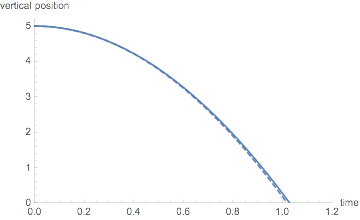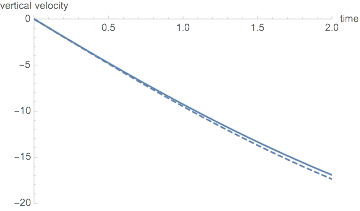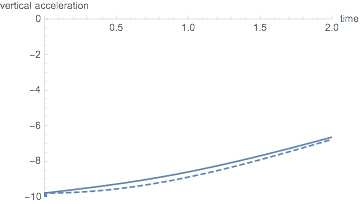The difference in all parameters is minimal.

Next, a firing speed near terminal velocity: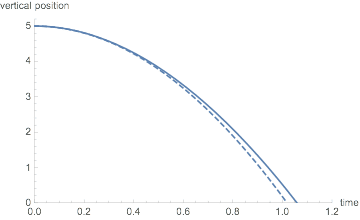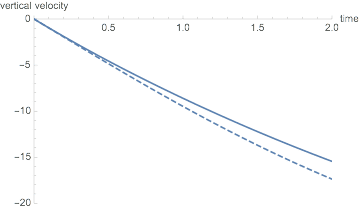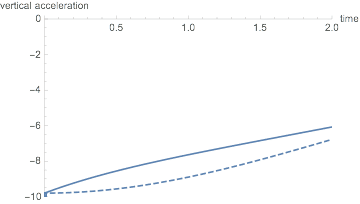Finally, for good measure, firing at roughly twice the terminal velocity: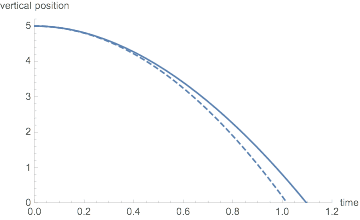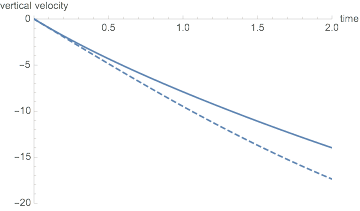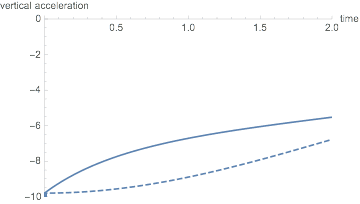Indeed, the greater the initial horizontal velocity the greater the impact on the vertical components of the motion.

#### Attachments

•ChessEnthusiast, Delta2 and PhanthomJay
rcgldr
Homework Helper
It might help to explain that when drag force is split up into components (parametric equations), you end up with something like:

$$drag_x = 1/2 \rho\ C_d\ v_x |v|$$
$$drag_y = 1/2 \rho\ C_d\ v_y |v|$$
$$|v| = \sqrt{v_x^2 + v_y^2}$$

Where |v| is the magnitude of the velocity, independent of direction. There isn't a closed form solution to this problem, so some type of numerical integration is used. Trying to find a source for these equations requires doing a search for parametric drag equation. Page 30 of this pdf file includes equations similar to what I wrote above.

••